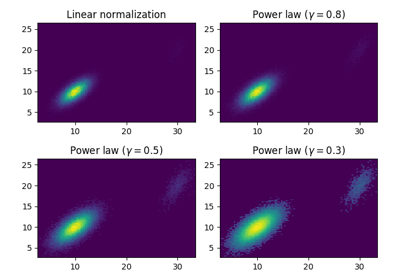/Matplotlib 3.1

# matplotlib.pyplot.hist2d

`matplotlib.pyplot.hist2d(x, y, bins=10, range=None, density=False, weights=None, cmin=None, cmax=None, *, data=None, **kwargs)` [source]

Make a 2D histogram plot.

Parameters: `x, y : array_like, shape (n, )` Input values `bins : None or int or [int, int] or array_like or [array, array]` The bin specification: If int, the number of bins for the two dimensions (nx=ny=bins). If `[int, int]`, the number of bins in each dimension (nx, ny = bins). If array_like, the bin edges for the two dimensions (x_edges=y_edges=bins). If `[array, array]`, the bin edges in each dimension (x_edges, y_edges = bins). The default value is 10. `range : array_like shape(2, 2), optional, default: None` The leftmost and rightmost edges of the bins along each dimension (if not specified explicitly in the bins parameters): ```[[xmin, xmax], [ymin, ymax]]```. All values outside of this range will be considered outliers and not tallied in the histogram. `density : bool, optional, default: False` Normalize histogram. normed is a deprecated synonym for this parameter. `weights : array_like, shape (n, ), optional, default: None` An array of values w_i weighing each sample (x_i, y_i). `cmin : scalar, optional, default: None` All bins that has count less than cmin will not be displayed and these count values in the return value count histogram will also be set to nan upon return `cmax : scalar, optional, default: None` All bins that has count more than cmax will not be displayed (set to none before passing to imshow) and these count values in the return value count histogram will also be set to nan upon return `h : 2D array` The bi-dimensional histogram of samples x and y. Values in x are histogrammed along the first dimension and values in y are histogrammed along the second dimension. `xedges : 1D array` The bin edges along the x axis. `yedges : 1D array` The bin edges along the y axis. `image : QuadMesh` `cmap : Colormap or str, optional` A `colors.Colormap` instance. If not set, use rc settings. `norm : Normalize, optional` A `colors.Normalize` instance is used to scale luminance data to `[0, 1]`. If not set, defaults to `colors.Normalize()`. `vmin/vmax : None or scalar, optional` Arguments passed to the `Normalize` instance. `alpha : 0 <= scalar <= 1 or None, optional` The alpha blending value.

`hist`
1D histogram plotting

#### Notes

• Currently `hist2d` calculates it's own axis limits, and any limits previously set are ignored.
• Rendering the histogram with a logarithmic color scale is accomplished by passing a `colors.LogNorm` instance to the norm keyword argument. Likewise, power-law normalization (similar in effect to gamma correction) can be accomplished with `colors.PowerNorm`.

Note

In addition to the above described arguments, this function can take a data keyword argument. If such a data argument is given, the following arguments are replaced by data[<arg>]:

• All arguments with the following names: 'weights', 'x', 'y'.

Objects passed as data must support item access (`data[<arg>]`) and membership test (`<arg> in data`).

## Examples using `matplotlib.pyplot.hist2d`Exploring normalizations